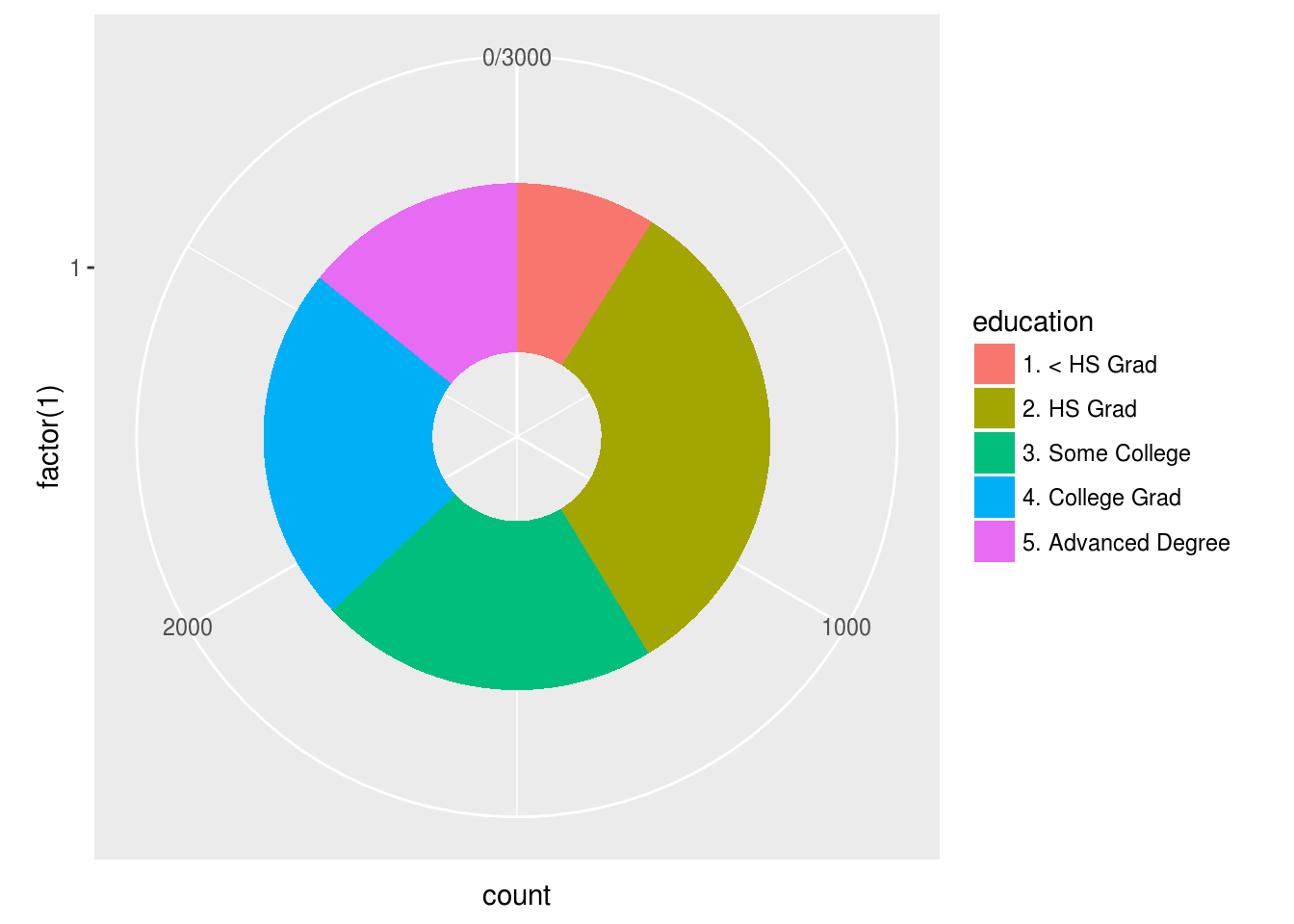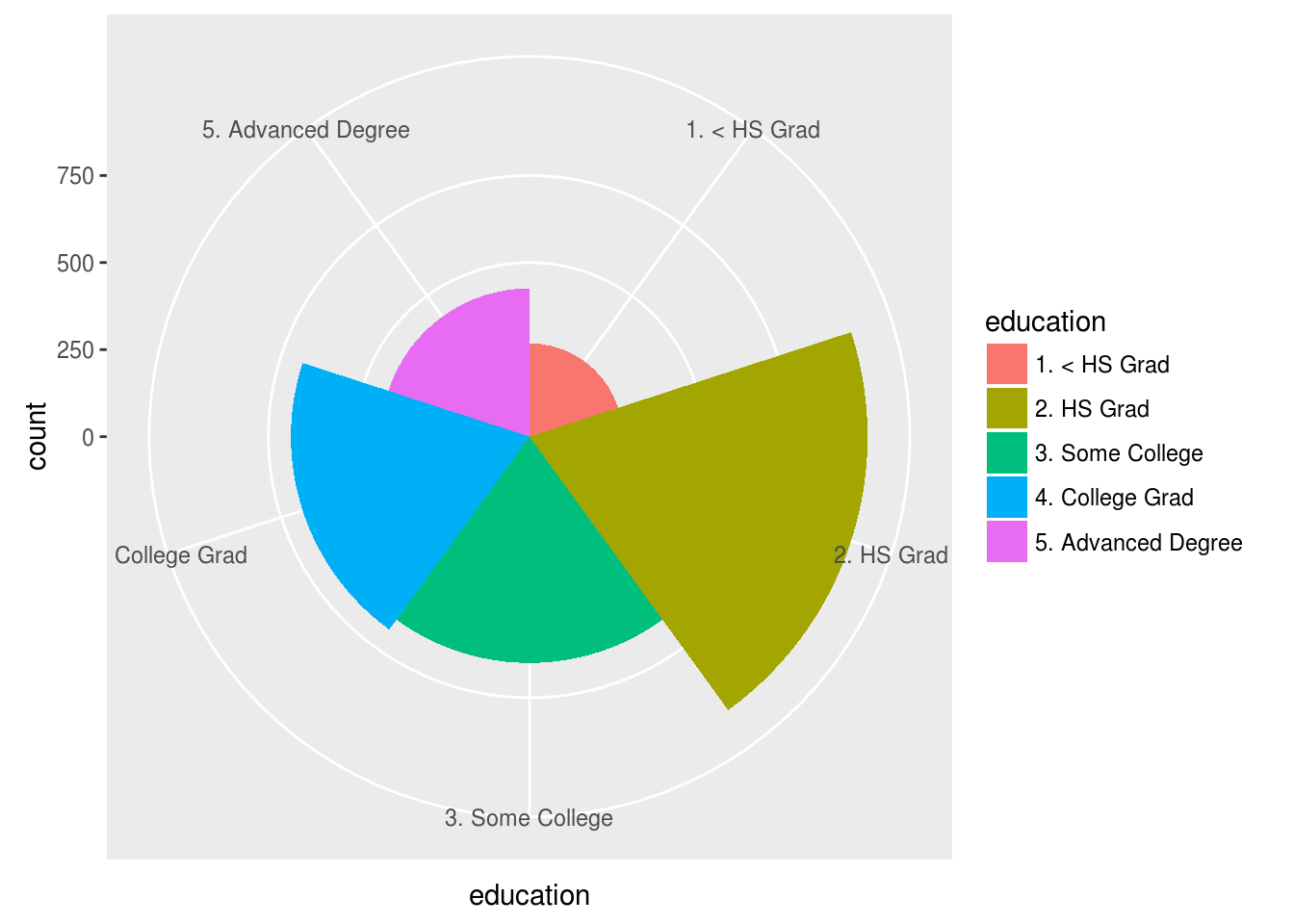# Pie Charts and More Using ggplot2

This post will explain several types of visuals that can be developed in using ggplot2. In particular, we are going to make three specific types of charts and they are…

• Pie chart
• Bullseye chart
• Coxcomb diagram

To complete this task, we will use the “Wage” dataset from the “ISLR” package.We will use the “education” variable which has five factors in it. Below is the initial code to get started.

``````library(ggplot2);library(ISLR)
data("Wage")``````

Pie Chart

In order to make a pie chart, we first need to make a bar chart and add several pieces of code to change it into a pie chart. Below is the code for making a regular bar plot.

``ggplot(Wage, aes(education, fill=education))+geom_bar()``We will now modify two parts of the code. First, we do not want separate bars. Instead, we want one bar. The reason being is that we only want one pie chart so before that we need one bar. Therefore, for the x value in the “aes” function, we will use the argument “factor(1)” which tells R to force the data as one factor on the chart thus making one bar. We also need to add the “width=1” inside the “geom_bar” function. This helps with spacing. Below is the code for this

``ggplot(Wage, aes(factor(1), fill=education))+geom_bar(width=1)``To make the pie chart, we need to add the “coord_polar” function to the code which adjusts the mapping. We will include the argument “theta=y” which tells R that the size of the pie a factor gets depends on the number of people in that factor. Below is the code for the pie chart.

``````ggplot(Wage, aes(factor(1), fill=education))+
geom_bar(width=1)+coord_polar(theta="y")``````By changing the “width” argument you can place a circle in the middle of the chart as shown below.

``````ggplot(Wage, aes(factor(1), fill=education))+
geom_bar(width=.5)+coord_polar(theta="y")``````Bullseye Chart

A bullseye chart is a pie chart that shares the information in a concentric way. The coding is mostly the same except that you remove the “theta” argument from the “coord_polar” function. The thicker the circle the more respondents within it. Below is the code

``````ggplot(Wage, aes(factor(1), fill=education))+
geom_bar(width=1)+coord_polar()``````Coxcomb Diagram

The Coxcomb Diagram is similar to the pie chart but the data is not normalized to fit the entire area of the circle. To make this plot we have to modify the code to make the by removing the “factor(1)” argument and replacing it with the name of the variable and be reading the “coord_polor” function. Below is the code

``````ggplot(Wage, aes(education, fill=education))+
geom_bar(width=1)+coord_polar()``````Conclusion

These are just some of the many forms of visualizations available using ggplot2. Which to use depends on many factors from personal preference to the needs of the audience.